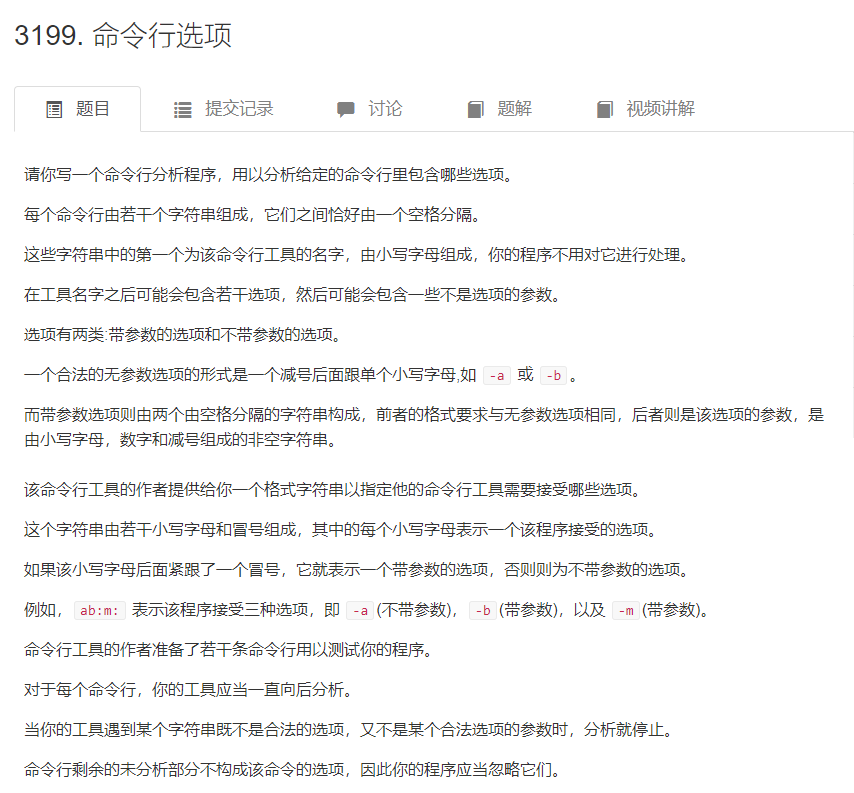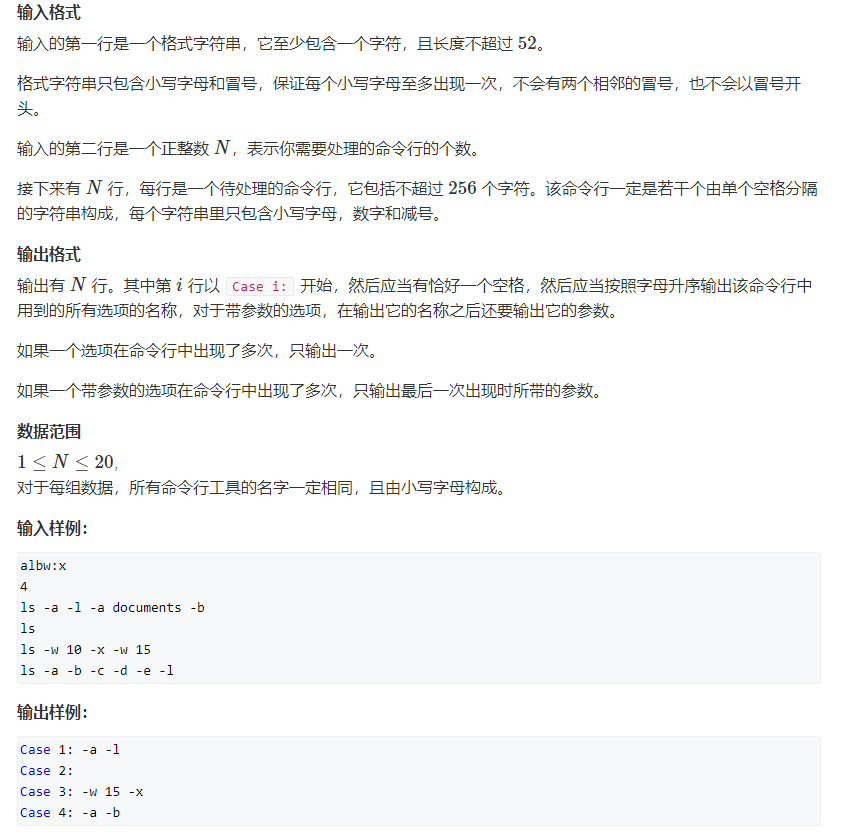# CSP认证201403-3 命令行选项2[C++题解]：模拟题、字符串处理、stringstream处理getline

• 时间:
• 浏览:

• 题目解答
• 题目链接

### 题目解答``````ls -a -l -a documents -b
```````````` stringstream  ssin(str);//从字符串开始读,以空格隔开
vector<string> ops;
while(ssin>>str) ops.push_back(str);
``````

ac代码

``````#include<bits/stdc++.h>
using namespace std;
const int N = 30;
int n;
bool o1[N], o2[N];// o1是无参数， o2是有参数
string ans[N]; //存答案

int main(){
string str;
cin >> str;
for(int i = 0; i< str.size(); i++)
if( i+ 1 < str.size() &&  str[i+1] == ':'){
o2[str[i] - 'a'] = true; // 有参数的
i++; // 跳过冒号
}
else o1[str[i] - 'a'] = true;  // 无参数的

cin >> n;
getchar();
for(int C = 1;C <= n; C++){
printf("Case %d:", C);
getline(cin, str);
stringstream ssin(str);
vector<string> ops;
while(ssin >> str) ops.push_back(str);
for(int i = 0; i < 26; i++)  ans[i].clear();
// 处理每个命令行选项
for(int i =1; i< ops.size(); i++){
if(ops[i] != '-' || ops[i] < 'a' || ops[i].size() != 2)
break;
int k = ops[i] - 'a'; //选项的编号
if(o1[k]) ans[k] = "*";
// 有参数的，ans[k]存的是参数值
else if( o2[k] &&  i + 1 < ops.size() ) ans[k] = ops[i+1], i ++;
else break;
}
// 按照字典序输出选项 a ~ z
for(int i = 0; i< 26; i++){
if( ans[i].size()){
cout << " -" << (char)(i + 'a');
if(o2[i]) cout << ' ' << ans[i];
}
}
cout << endl;

}
}
``````

### 题目链接

https://www.acwing.com/problem/content/3202/